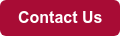This blog post is courtesy of European Sensor Systems (ES Systems), a provider of highly accurate pressure sensors and mass flow sensors.

How do you convert an air velocity measurement to a volumetric flow rate? It is important to understand the various formulas and technical definitions to prevent errors in calculation.

Volumetric flow rate is the volume of fluid that passes per unit of time.

Flow meter technologies such as differential pressure, magnetic, thermal, turbine, ultrasonic, and vortex all measure flow rate as a function of fluid velocity. Please refer to the respective flow meter datasheet for detailed information and specifications.

You can calculate the volumetric flow rate by using the equation shown below:

volumetric Flow Rate (Q) = Flow Velocity (V) × Cross-sectional Area (A)

Where,

Q is the Volumetric Flow Rate in m3/s

V is the Flow Velocity in m/s

A is the cross-sectional area in mm2For example, if a gas had a velocity (V) 15 m/s and was traveling through a pipe of 20mm inner diameter then the volumetric Flow Rate (Q) would be 0.004712 m3/s which is equal to 282.74 l/min.

## Converting velocity to mass flow rate

Similarly, the volumetric flow rate can be converted to the mass flow rate if the density of the gas measured is known.Where,

ṁ is the mass flow rate in kg/s

ρ is the density in kg/m3

From the two equations above it can be derived that:

Mass Flow Rate (ṁ) = V × A × ρ

Using the same example as above, if the density was 998 kg/m3 then the volumetric flow rate of 282.74 l/min would be equivalent to a mass flow rate of 4.703 kg/s.

## Velocity profiles – Laminar & turbulent flow

Laminar flow is described as fluid particles following smooth paths in layers, with each layer flowing smoothly past the adjacent layers with little to no mixing in fluid dynamics. The fluid continues to flow without lateral mixing at low velocities, and neighboring layers float past each other like playing cards.

Turbulence, also known as turbulent flow, is a fluid motion characterized by chaotic variations in pressure and flow rate in fluid mechanics.Laminar flow can be, in general, achieved by providing a 20x the internal diameter of the tube used straight tube length in upstream and downstream flow. For example, if a 25mm ID tube is used, if you connect a 50cm straight tube upstream and downstream of the flow meter, you can expect a laminar flow.

In most applications, turbulent flow is present in the application. This increases the flow noise observed by the flow meter.

## Volumetric & mass flow rate units

Volumetric flow rate can be expressed in a variety of units with the most common being m3/s, m3/min, m3/h in metric units and the respective imperial units. Volumetric flow rate is also typically expressed in l/s, l/min, l/h.

Mass flow rate is usually referenced in kg/s, kg/min, kg/h in metric units and in lb/s, lb/min, lb/h in the respective imperial units. Mass flow rate can be also expressed in l/s, l/min, l/h but with one condition.

Due to the fact that for mass flow measurement the gas density must be taken into account, when using l/min unit for mass flow, the temperature and pressure reference conditions must be noted.

There are two common reference conditions for mass flow measurements. These are:

• Normal, expressed at 0oC temperature and 1013 mbar pressure and denoted as ln/s, ln/min, ln/h
• Standard, expressed at 20oC temperature and 1013 mbar pressure and denoted as ls/s, ls/min, ls/h

Converting mass flow from reference to ambient conditions can be achieved using the following formula:Where:

• Pressure is in mbar
• Temperature is in Kelvin

## Converting differential pressure to flow velocity

Before going into how to convert differential pressure to flow velocity, it is important to note the definitions of static, dynamic, and total pressure.

What’s pressure?

The constant physical force exerted on or against an object by something (such as air) in contact with it is known as pressure.

What’s static pressure?

The pressure you get when the fluid isn’t flowing or when you’re moving with the fluid is called static pressure. While now moving, air would press against you equally in all directions. Because of the conservation law, static pressure decreases as the speed increases.

What’s total pressure?

The pressure a fluid exerts when it comes to a full stop is known as absolute (or total) pressure. When you face the wind and the air collides with your body, total pressure acts on you.

What’s dynamic pressure?

Dynamic pressure is the pressure exerted by a fluid as it travels. It refers to the difference between total and static pressure.

In order to measure flow via differential pressure, a deliberate obstacle has to be introduced in the flow line in order to impose the increased differential pressure measurement. The obstacle can be anything, from an orifice to a simple narrowing of the tube.

Using Bernoulli’s principle, you can correlate flow velocity with differential pressure using the following formula:## Measuring velocity and airflowESCP-BMS1 ESRF-ESF ESRF-HF

• ESCP-BMS1 – a series of board mountable pressure sensors for applications requiring high resolution and accuracy. Total error band of 0.25% FS from 0-60C.
• ESRF-ESF – an inline gas flow sensor based on the hot-film anemometer principle for mass gas flow measurements.
• ESRF-HF – a family of insertion gas flow transmitters based on the hot-film anemometer principle for mass gas flow measurements.

We are here to answer further questions you may have. Contact us to tell us what you are looking for!